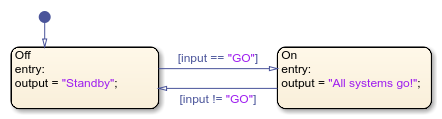## Manage Textual Information by Using Strings

You can control chart behavior and output easy-to-read text by using strings to create textual data.

### Creating Strings in Stateflow

In Stateflow®, a string is a piece of text surrounded by double quotes (`"`...`"`). In addition, single-quoted strings (`'`...`'`) are supported in charts that use C as the action language. For example, this chart takes string data as input. Based on that input, the chart produces a corresponding string output.To specify a string symbol, first open the Property Inspector. In the Symbols pane, select the data that you want to convert to a string. In the Property Inspector, set the Type to `string`. Stateflow dynamically allocates memory space for this type of data.

### Computation with Strings

To manipulate string data in a Stateflow chart, use the operators in this table.

GoalMATLAB Action Language FunctionExampleC Action Language FunctionExample

Concatenate two strings

`plus`
```h = "Hello," w = " world!" x = plus(h,w) ```
`strcat`
```s1 = "State"; s2 = "flow"; dest = strcat(s1,s2);```

Determine the length of a string

`strlength`
```h = "Hello, world!" x = strlength(h)```
`strlen`
`L = strlen("Stateflow");`

Convert a string to a double

`str2double`
`X = str2double("-12.345");`
`str2double`
`X = str2double("-12.345");`

Convert numeric, Boolean, or enumerated data to string

`string`
```a = ; str = string(a)```
`tostring`
`dest = tostring(RED);`

### String Truncation

You can also create string data with a maximum number of characters. To specify symbol as a string with a buffer size of `n` characters, set the Type field of the symbol to `stringtype(n)`. The text of the string can be shorter than the buffer, but if the string length exceeds the buffer size, then the text in the string is truncated. For example, if the Type property of the symbol `output` in the previous chart is `stringtype(10)`, then its value in the state `On` is truncated to `"All system"`.

You can enable the String truncation checking parameter to choose whether to stop simulation or generate a warning when a string exceeds the length specified by `stringtype(n)`.

String Truncation CheckingDescription
`error`Simulation stops with an error.
`warning`String is truncated. Simulation continues with a warning.
`none`String is truncated. Simulation continues with no error or warning.

Note

Unlike C or C++, Stateflow interprets escape sequences as literal characters. For example, the string `"\n"` contains two characters, backslash and `n`, and not one newline character.

### Differences Between Charts That Use MATLAB and C as the Action Language

Key differences between strings in charts that use C as the action language and charts that use MATLAB® include:

• Charts that use MATLAB as the action language support only strings enclosed with double-quotes. In charts that use C as the action language, strings can use double quotes or single quotes.

• In charts that use MATLAB as the action language,`strcmp` returns `1 (true)` when the strings match. In charts that use C as the action language, `strcmp` returns `0`.

• In charts that use MATLAB as the action language, `strcmp` returns a boolean. In charts that use C as the action language, `strcmp` returns a double.

• To return the length of the string, use `strlength` in charts that use MATLAB as the action language and `strlen` in charts that use C as the action language.

• To concatenate a string, use the `+` operation in charts that use MATLAB as the action language and `strcat` in charts that use C as the action language.

• Charts that use MATLAB as the action language enforce complexity on the output variable when using `str2double`.

• Charts that use MATLAB as the action language support all comparison operations, such as `>`, `<`, or `==`.

### Limitations

Parameter data cannot be strings.

The following limitations exist for charts that use MATLAB as the action language:

• Constant data cannot be strings.

• These operators are not supported:

• `strcat`

• `extract`

• `extractBetween`

• `sscanf`

• `compose`

• `append`

• `pad`

• `count`

• `sfprint`

• Structures can only use these operators:

• `isstring`

• `strcmp`

• `string`

• `strlength`